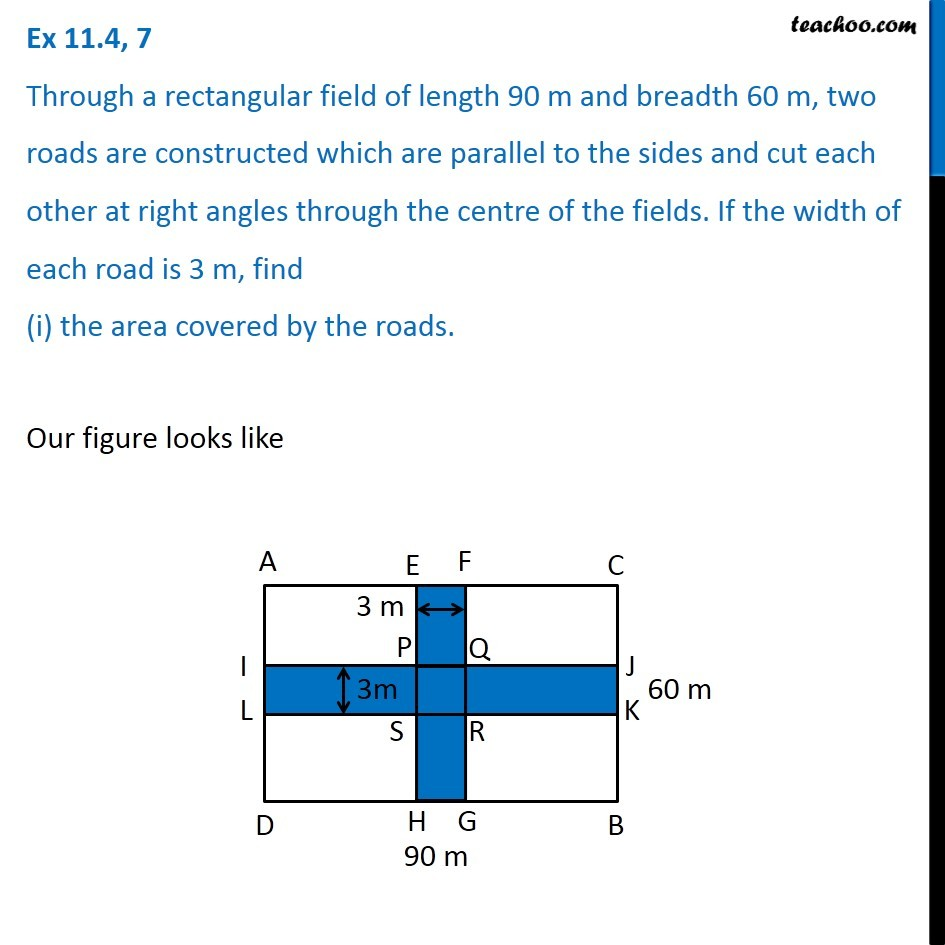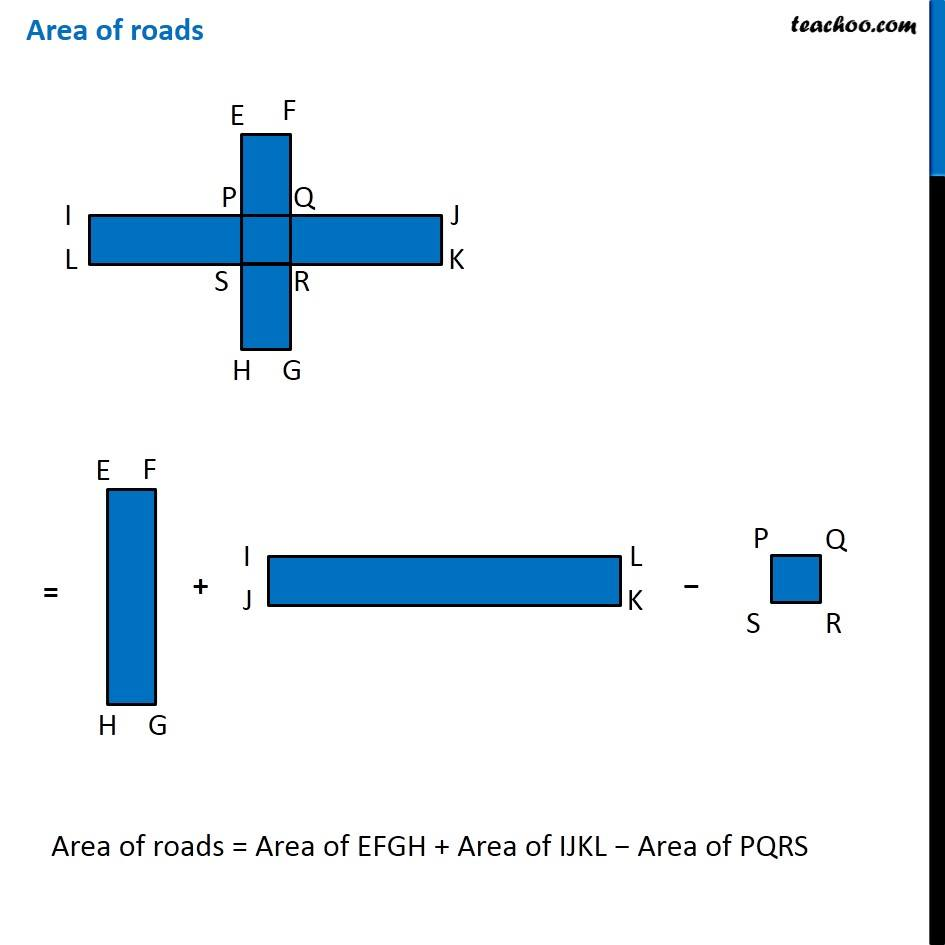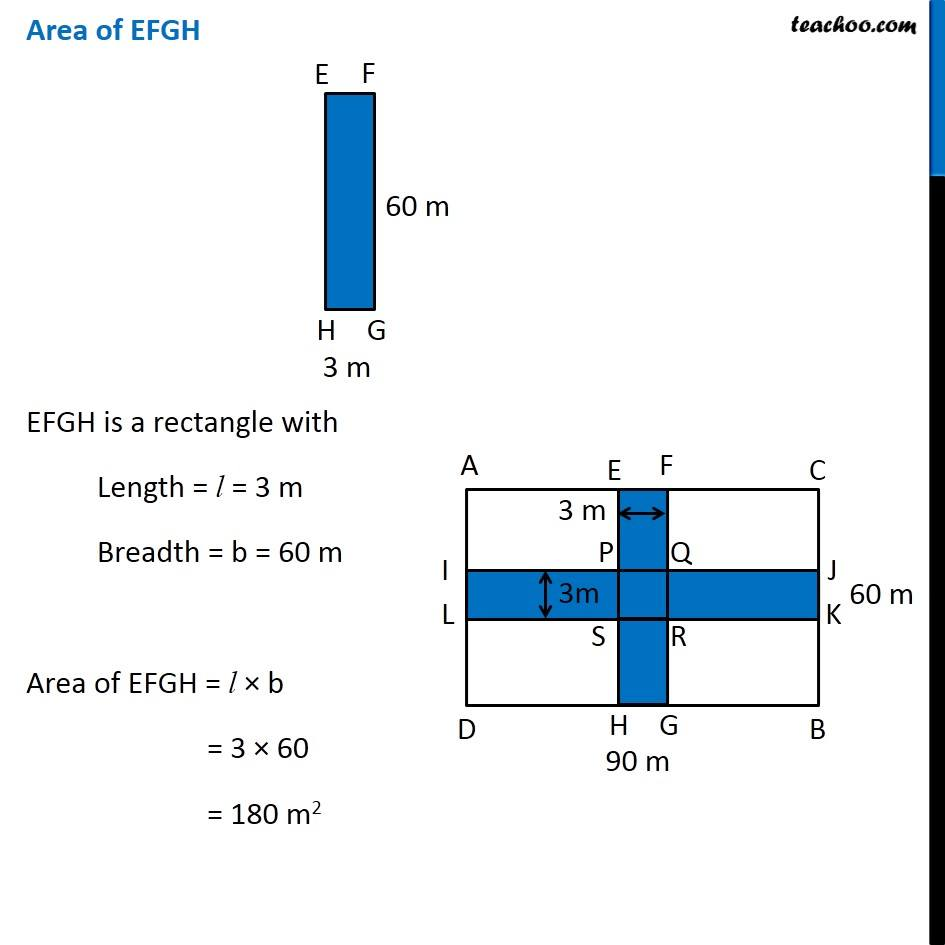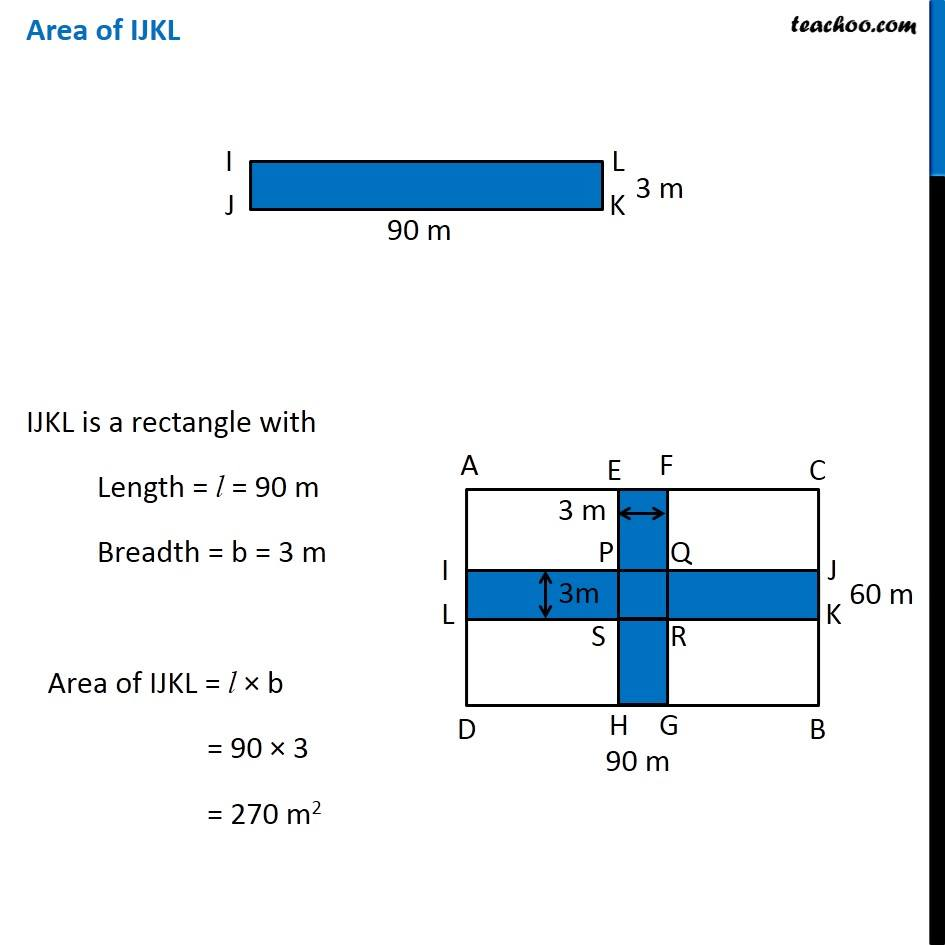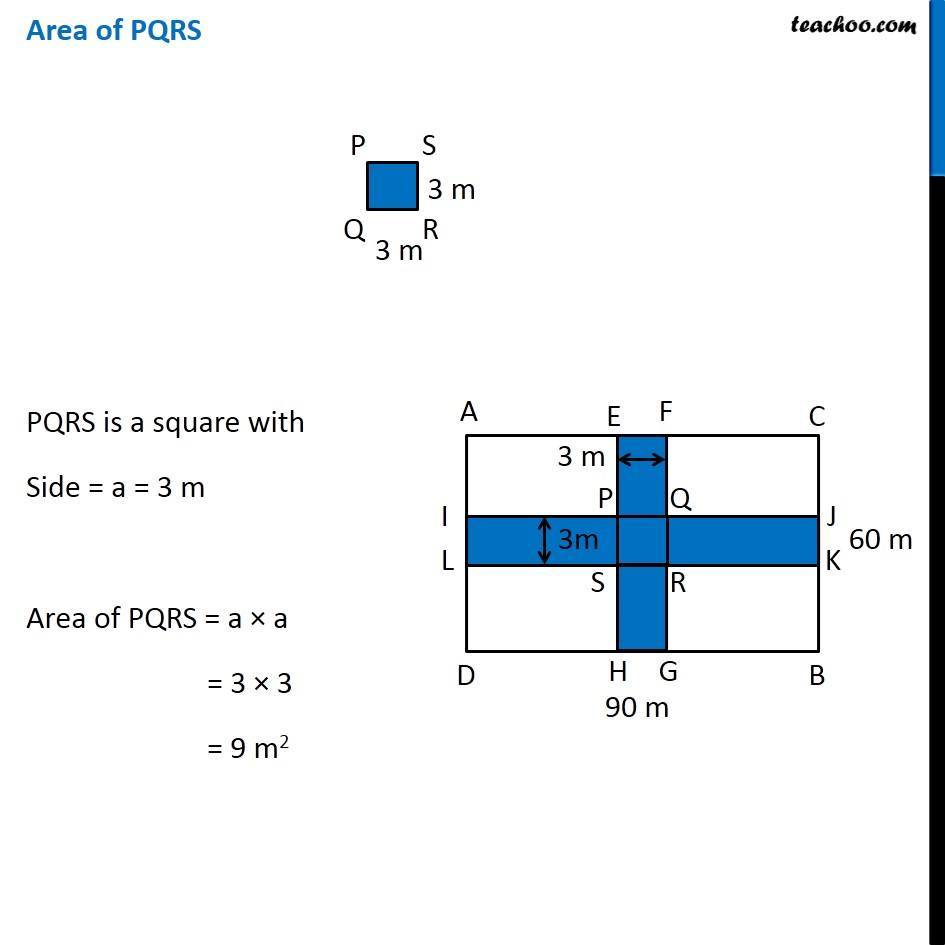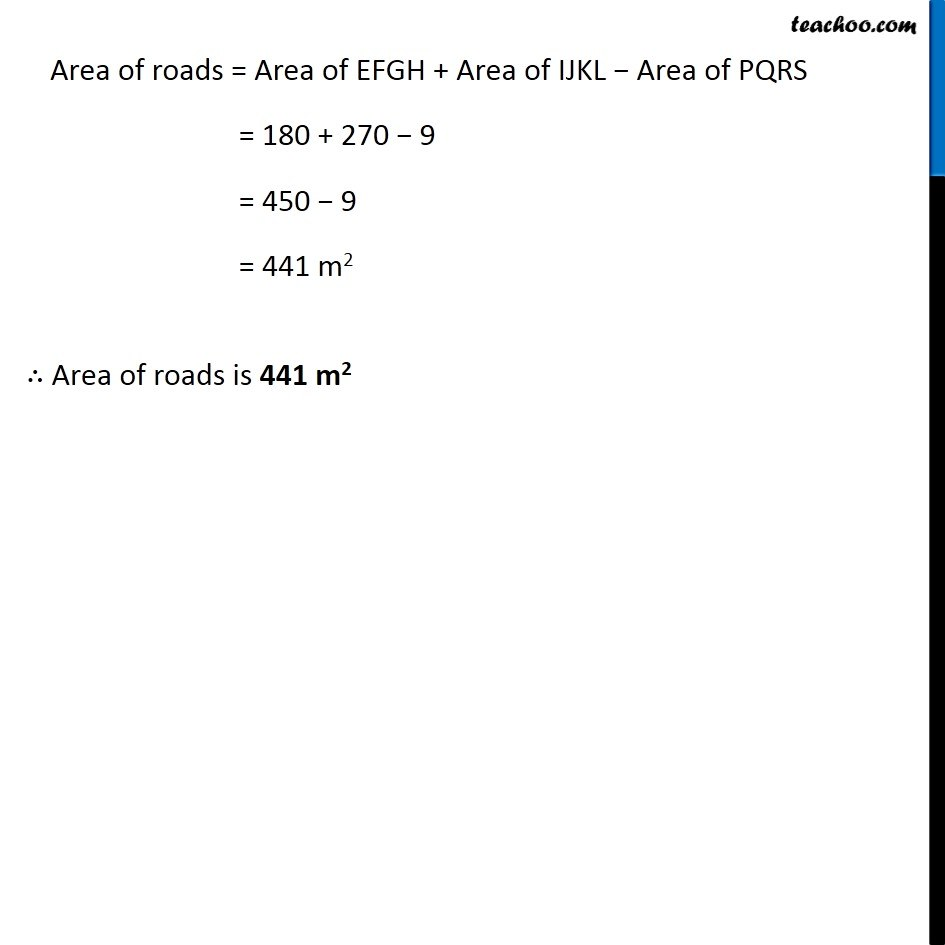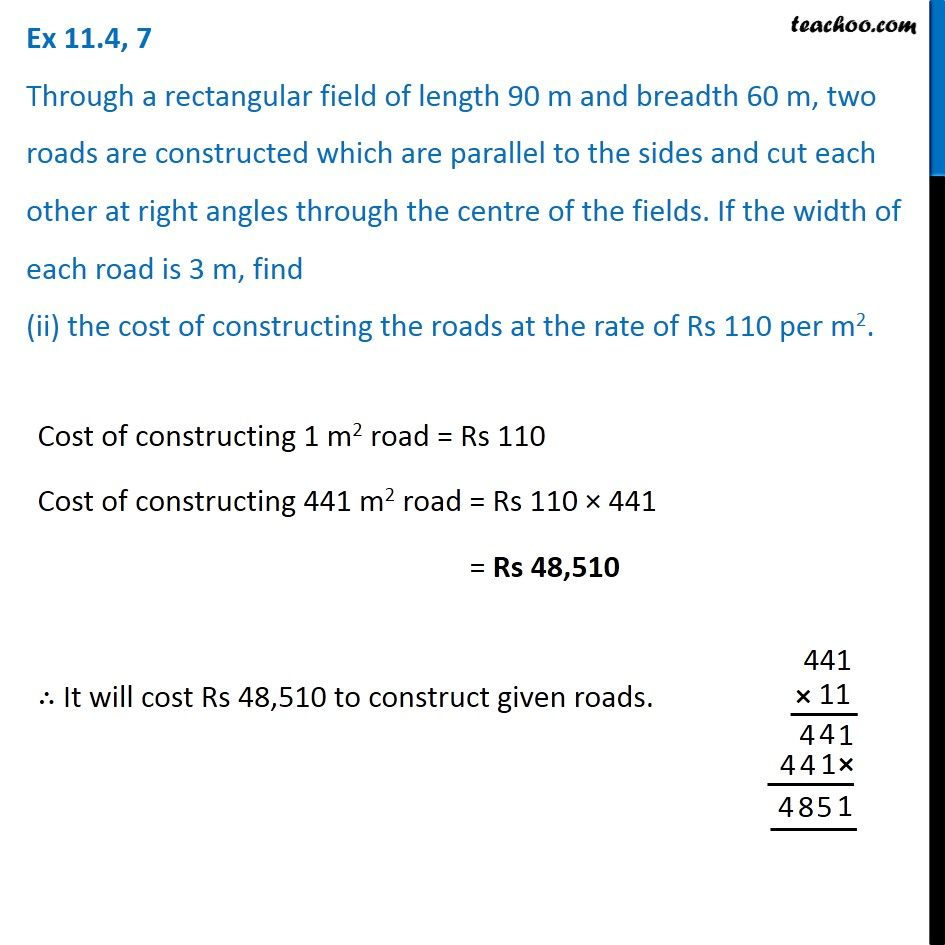1. Chapter 11 Class 7 Perimeter and Area
2. Concept wise
3. Finding Area of rectangle with cross roads

Transcript

Ex 11.4, 7 Through a rectangular field of length 90 m and breadth 60 m, two roads are constructed which are parallel to the sides and cut each other at right angles through the centre of the fields. If the width of each road is 3 m, find (i) the area covered by the roads. Our figure looks like Area of roads Area of roads = Area of EFGH + Area of IJKL − Area of PQRS Area of EFGH EFGH is a rectangle with Length = l = 3 m Breadth = b = 60 m Area of EFGH = l × b = 3 × 60 = 180 m2 Area of IJKL IJKL is a rectangle with Length = l = 90 m Breadth = b = 3 m Area of IJKL = l × b = 90 × 3 = 270 m2 Area of PQRS PQRS is a square with Side = a = 3 m Area of PQRS = a × a = 3 × 3 = 9 m2 Area of roads = Area of EFGH + Area of IJKL − Area of PQRS = 180 + 270 − 9 = 450 − 9 = 441 m2 ∴ Area of roads is 441 m2 Ex 11.4, 7 Through a rectangular field of length 90 m and breadth 60 m, two roads are constructed which are parallel to the sides and cut each other at right angles through the centre of the fields. If the width of each road is 3 m, find (ii) the cost of constructing the roads at the rate of Rs 110 per m2. Cost of constructing 1 m2 road = Rs 110 Cost of constructing 441 m2 road = Rs 110 × 441 = Rs 48,510 ∴ It will cost Rs 48,510 to construct given roads.

Finding Area of rectangle with cross roads

About the AuthorDavneet Singh
Davneet Singh is a graduate from Indian Institute of Technology, Kanpur. He has been teaching from the past 10 years. He provides courses for Maths and Science at Teachoo.